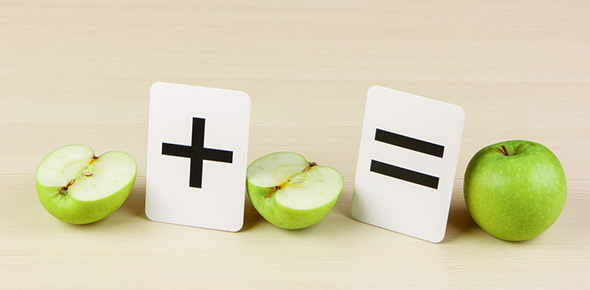# Fractions And Percentages

7 QuestionsSettings.

Related Topics
• 1.
Fraction is part of a whole.
• A.

True

• B.

False

• 2.
Is Mr. Bulut's solution correct? 1/2 + 1/2 = 2/4
• A.

Correct

• B.

Wrong

• 3.
What is 1/2 + 1/4?
• A.

2/5

• B.

1/3

• C.

3/4

• D.

1/8

• 4.
What is 3/4 + 1/8?
• A.

7/8

• B.

4/12

• C.

3/32

• D.

3/12

• 5.
How do you calculate 20% of 80?
• A.

I convert 20% into decimal which is 0.20, then multiply by 80.

• B.

I convert 20% into fraction 20/100, then multiply by 80.

• C.

I don't do anything. The answer is 20.

• 6.
What is 20% of 120?
• A.

24

• B.

48

• C.

96

• D.

2400

• 7.
Mr. Bulut scored 70 out of 90. Did he pass his driving test? (NOTE: passing mark is 80%)
• A.

Passed

• B.

Failed